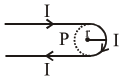# A hairpin like shape

Question:

A hairpin like shape as shown in figure is made by bending a long current carrying wire. What is the magnitude of a magnetic field at point $P$ which lies on the centre of the semicircle ?1. $\frac{\mu_{0} \mathrm{I}}{4 \pi \mathrm{r}}(2-\pi)$

2. $\frac{\mu_{0} \mathbf{I}}{4 \pi \mathrm{r}}(2+\pi)$

3. $\frac{\mu_{0} \mathbf{I}}{2 \pi \mathrm{r}}(2+\pi)$

4. $\frac{\mu_{0} \mathbf{I}}{2 \pi r}(2-\pi)$

Correct Option: , 2

Solution:

(2) $\mathrm{B}=2 \times \mathrm{B}_{\text {st.wire }}+\mathrm{B}_{\text {loop }}$

$\mathrm{B}=2 \times \frac{\mu_{0} \mathrm{i}}{4 \pi \mathrm{r}}+\frac{\mu_{0} \mathrm{i}}{2 \mathrm{r}}\left(\frac{\pi}{2 \pi}\right)$

$\mathrm{B}=\frac{\mu_{0} \mathrm{i}}{4 \pi \mathrm{r}}(2+\pi)$